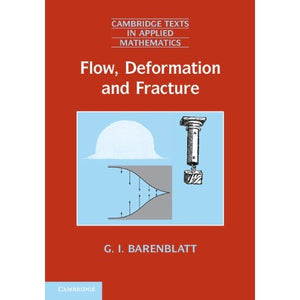># Flow, Deformation and Fracture: Lectures on Fluid Mechanics and the Mechanics of Deformable Solids for Mathematicians and Physicists: 49 (Cambridge Texts in Applied Mathematics, Series Number 49)

• £10.89
• Save £17

Grigory Isaakovich Barenblatt
Cambridge University Press, 6/5/2014
EAN 9780521715386, ISBN10: 0521715385

Paperback, 273 pages, 24.6 x 17.5 x 1.5 cm
Language: English

Over forty years of teaching experience are distilled into this text. The guiding principle is the wide use of the concept of intermediate asymptotics, which enables the natural introduction of the modeling of real bodies by continua. Beginning with a detailed explanation of the continuum approximation for the mathematical modeling of the motion and equilibrium of real bodies, the author continues with a general survey of the necessary methods and tools for analyzing models. Next, specific idealized approximations are presented, including ideal incompressible fluids, elastic bodies and Newtonian viscous fluids. The author not only presents general concepts but also devotes chapters to examining significant problems, including turbulence, wave-propagation, defects and cracks, fatigue and fracture. Each of these applications reveals essential information about the particular approximation. The author's tried and tested approach reveals insights that will be valued by every teacher and student of mechanics.

Foreword Alexandre J. Chorin
Preface
Introduction
1. Idealized continuous media
the basic concepts
2. Dimensional analysis and physical similitude
3. The ideal incompressible fluid approximation
general concepts and relations
4. The ideal incompressible fluid approximation
analysis and applications
5. The approximation of a linear elastic solid. Basic equations and boundary value problems of linear theory of elasticity
6. Approximation of a linear elastic body. Applications
brittle and quasi-brittle fracture, strength of structures
7. The approximation of Newtonian viscous fluids